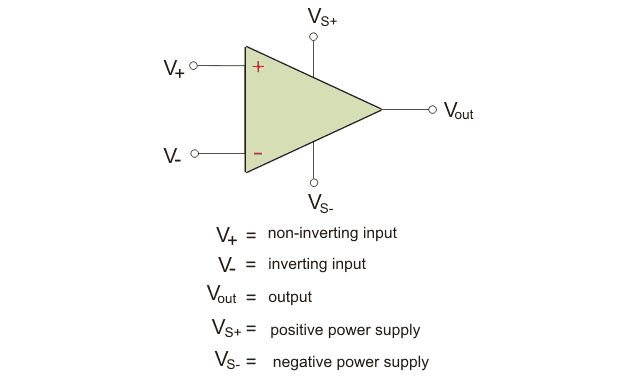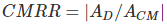# Op Amp Characteristics

Operational amplifier or op amps as they are usually referred are linear devices that can give ideal DC amplification. They are fundamentally voltage amplifying devices used with external feedback components like resistors or capacitors. An op amp is a three terminal device, with one terminal called the inverting input, other the non-inverting input and the last one is the output. Below is a diagram of a typical op amp:As you can see from the diagram, op amp has three terminals for input and output and 2 for power supply.
Before we understand the operation of an op amp, we must learn about the op amp characteristics of an op amp. We will explain them one by one here:

### Open Loop Voltage Gain(A)

The open loop voltage gain without any feedback for an ideal op amp is infinite. But typical values of open loop voltage gain for a real op amp ranges from 20,000 to 2, 00,000. Let the input voltage be Vin. Let A be the open loop voltage gain. Then the output voltage is Vout = AVin. The value of a typically is in the range specified above but for an ideal op amp, it is infinite.

### Input Impedance(Zin)

Input Impedance is defined as the input voltage by the input current. The input impedance of an ideal op amp is infinite. That is there no current flowing in the input circuit. However, an ideal op amp has certain current flowing in the input circuit of the magnitude of few pico-amps to a few milli-amps.

### Output Impedance (Zout)

Output impedance is defined as the ratio of the output voltage to the input current. The output impedance of an ideal op amp is zero, however, real op amps have an output impedance of 10-20 kΩ. An ideal op amp behaves like a perfect voltage source delivering current without any internal losses. The internal resistance reduce the voltage available to the load.

### Bandwidth(BW)

An ideal op amp has an infinite bandwidth that is it can amplify any signal from DC to the highest AC frequencies without any losses. So therefore, an ideal op amp is said to have infinite frequency response. In real op amps, the bandwidth is generally limited. The limit depends on the gain bandwidth (GB) product. GB is defined as the frequency where the amplifier gain becomes unity.

### Offset Voltage(Vio)

The offset voltage of an ideal op amp is zero, which means that the output voltage will be zero if the difference between the inverting and non-inverting terminal is zero. If both the terminals are grounded, the output voltage will be zero. But real op amps have an offset voltage.

### Common Mode Rejection Ratio(CMRR)

Common mode refers to the situation when the same voltage is applied to both the inverting and non-inverting terminal of the op amp. The common mode rejection refers to the ability of the op amp to reject the common mode signal. Now we are in a position to understand the term common mode rejection ratio.
The common mode rejection ratio refers to the measure of the ability of the op amp to reject the common mode signal. Mathematically it is defined asWhere, AD is the differential gain of the op amp, ∞ for an ideal op amp.
ACM refers to the common mode gain of the op-amp.
The CMRR of an ideal op amp is ∞. That means it is able to reject all common mode signal. Also from the formula, we can see the AD is infinite for an ideal op amp and ACM is zero. Therefore the CMRR of an ideal op-amp is infinite. Therefore it will reject any signal which is common to both.
However, real omp have finite CMRR, and does not reject all common mode signals.

Want To Learn Faster? 🎓
Get electrical articles delivered to your inbox every week.
No credit card required—it’s 100% free.